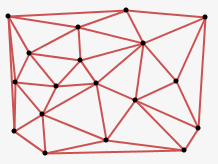#### Howdy, Stranger!

We are about to switch to a new forum software. Until then we have removed the registration on this forum.

# Need help to fix a bug in the code (triangle packing)

edited September 2017

Here is the sketch with triangles appears one from the other. Triangles should NOT appear in the place where there already is a triangle (the strings of code doing this is for example 41, 46, 48), but they appear, the different opacity of different triangles shows that many triangles is on one place. How to make triangles NOT appear two or more times in the same place? Why my code not working properly?

``````ArrayList <Triangle> tris = new ArrayList <Triangle>();

color colore;
int step =10;

int firstx;
int firsty;

void setup()
{
size(800,800);
background(200);
colorMode(HSB);
colore=color(255,255,128);

firstx = 400;
firsty = 400;

PVector a = new PVector(firstx,firsty);
PVector b = new PVector(a.x+step/2,a.y+step);
PVector c = new PVector(b.x-step,b.y);

Triangle t1 = new Triangle (a,b,c,colore);
tris.add(t1);
}

void draw()
{
background(200);

int tr = int(random(tris.size()));

if(tris.get(tr).straight==true)
{
float rand = random(100);

if(rand>0&&rand<33)
{
if(tris.get(tr).ab==false)
{
PVector b=tris.get(tr).A.copy();
PVector a=tris.get(tr).B.copy();
PVector c=new PVector(b.x+step,b.y);
tris.get(tr).ab=true;
Triangle neww = new Triangle(a,b,c,colore);
neww.ab=true;
tris.add(neww);
}
}
else if(rand>33&&rand<66)
{
if(tris.get(tr).ac==false)
{
PVector c=tris.get(tr).A.copy();
PVector a=tris.get(tr).C.copy();
PVector b=new PVector(c.x-step,c.y);
tris.get(tr).ac=true;
Triangle neww = new Triangle(a,b,c,colore);
neww.ac=true;
tris.add(neww);
}
}
else
{
if(tris.get(tr).bc==false)
{
PVector b=tris.get(tr).C.copy();
PVector c=tris.get(tr).B.copy();
PVector a=new PVector(b.x+step/2,b.y+step);
tris.get(tr).bc=true;
Triangle neww = new Triangle(a,b,c,colore);
neww.bc=true;
tris.add(neww);
}
}
}

else
{
float rand = random(100);

if(rand>=0&&rand<33)
{
if(tris.get(tr).ab==false)
{
PVector b=tris.get(tr).A.copy();
PVector a=tris.get(tr).B.copy();
PVector c=new PVector(b.x-step,b.y);
tris.get(tr).ab=true;
Triangle neww = new Triangle(a,b,c,colore);
neww.ab=true;
tris.add(neww);
}
}
else if(rand>=33&&rand<66)
{
if(tris.get(tr).ac==false)
{
PVector c=tris.get(tr).A.copy();
PVector a=tris.get(tr).C.copy();
PVector b=new PVector(c.x+step,c.y);
tris.get(tr).ac=true;
Triangle neww = new Triangle(a,b,c,colore);
neww.ac=true;
tris.add(neww);
}
}
else
{
if(tris.get(tr).bc==false)
{
PVector b=tris.get(tr).C.copy();
PVector c=tris.get(tr).B.copy();
PVector a=new PVector(b.x-step/2,b.y-step);
tris.get(tr).bc=true;
Triangle neww = new Triangle(a,b,c,colore);
neww.bc=true;
tris.add(neww);
}
}
}

for(int i=0;i<tris.size();i++)
{
tris.get(i).display();
}
}

class Triangle
{
PVector A;
PVector B;
PVector C;

boolean ab=false;
boolean ac=false;
boolean bc=false;

boolean straight;
color colore1;

Triangle(PVector Ain,PVector Bin,PVector Cin, color colore1IN)
{
A = Ain;
B = Bin;
C = Cin;
colore1=colore1IN;

if(A.y<B.y) straight=true;
else straight=false;
}

void display()
{
fill(colore1,50);
noStroke();
beginShape(TRIANGLES);
vertex(A.x, A.y);
vertex(B.x, B.y);
vertex(C.x, C.y);
endShape();
}
}
``````
Tagged:

## Answers

• (please try to give your questions decent titles. just 'i need help' is obvious and useless. imagine if everyone posted like that...)

• @koogs, okay, I will

• Before placing a potential new triangle you need to check it against All other already placed triangles in a proper for loop

Only when none of them is in or near we are allowed to place a new triangle

• please explain your current algorithm. some comments in the code wouldn't go amiss...

what are the three options chosen by random? what is straight in line 35 and what does it do?

• The triangles creating one from another. Triangle has three sides - ab, ac, bc. I made three booleans ab, ac, bc, they are false by default, it means that new triangle can be created using one of these three sides. When new additional triangle is created, the corresponding side of old and new triangle changes to true, that means no triangle can be created using this side now. So, if triangle has ab=true, ac=true, bc = true, no new triangle can be created from this triangle. Suddenly, when I wrote this, I understand, why my code is wrong))) Many triangles appears in the same place not in the moment of birth, but after that, when the tree of triangles getting bigger.

• @Chrisir, you're right!

• "new triangle can be created using one of these three sides" -- this doesn't sound quite like packing -- more like space-filling. It sounds like you are subdividing the space into a random triangular mesh by adding points -- creating a Delaunay Diagram as in the Processing mesh library....The mesh way of doing this is to just pick all your points at random, then connect them into triangles, instead of drawing your starting triangle and then adding your random points one at a time.

Edit:

Ah, looking at your actual sketch I see that it is regular, not random.

• Re:

Many triangles appears in the same place not in the moment of birth, but after that, when the tree of triangles getting bigger.

Yes. Instead of a tree of triangles, you could use a square array to track your triangular grid. See the triangle grid examples here:

• this doesn't sound quite like packing

it was me that added that to the title, in an attempt to combat the vagueness.

• edited September 2017

@jeremydouglass , thanks for link.

Sign In or Register to comment.